2019-05-29 12:11:35 WHU_StudentZhong 阅读数 412
• ###### 用OpenStack和Kubernetes构建容器和虚拟机组合云平台

用OpenStack和Kubernetes构建容器和虚拟机组合云平台视频教程：1.集群的创建 2.容器和虚拟机网络问题 3.统一的身份认证 4.轻元科技在k8s上的创新和容器的存储方案

5829 人正在学习 去看看 CSDN讲师

（环境：VS2017+OPENCV4.0）

K值聚类算法并不复杂，这里不多介绍，直接上代码。

#include "pch.h"
#include <iostream>
#include <opencv2/opencv.hpp>
#include <math.h>
using namespace std;
using namespace cv;

struct SPoint {//点和点的类别
double v1;
double v2;
double v3;
int id;
};
void Color(SPoint* pt, int id) {//给不同类别赋予颜色，可以自己加
switch (pt->id) {
case 0:
pt->v1 = 0;
pt->v2 = 255;
pt->v3 = 170;
break;
case 1:
pt->v1 = 255;
pt->v2 = 0;
pt->v3 = 0;
break;
case 2:
pt->v1 = 0;
pt->v2 = 255;
pt->v3 = 0;
break;
case 3:
pt->v1 = 0;
pt->v2 = 0;
pt->v3 = 255;
break;
case 4:
pt->v1 = 255;
pt->v2 = 170;
pt->v3 = 200;
break;
case 5:
pt->v1 = 100;
pt->v2 = 100;
pt->v3 = 100;
break;
case 6:
pt->v1 = 200;
pt->v2 = 200;
pt->v3 = 200;
break;
}
}
double Norm2(SPoint p1, SPoint p2) {//距离的平方
return (pow((p1.v1 - p2.v1), 2) + pow((p1.v2 - p2.v2), 2) + pow((p1.v3 - p2.v3), 2));
//return (p1.v1 - p2.v1)*(p1.v1 - p2.v1) + (p1.v2 - p2.v2)*(p1.v2 - p2.v2) + (p1.v3 - p2.v3)*(p1.v3 - p2.v3);
}
Mat Kmean(Mat src, int species, int iterations) {//原图像，种类数，迭代次数
Mat K = src;
int  rows = src.rows, cols = src.cols;//行，列
SPoint* Points = new SPoint[rows*cols];
SPoint* CenterPt1 = new SPoint[species];
SPoint* CenterPt2 = new SPoint[species];
int* num = new int[species];
double* sum1 = new double[species];
double* sum2 = new double[species];
double* sum3 = new double[species];
for (int x = 0; x < cols; x++) {//把图像信息读入
for (int y = 0; y < rows; y++) {
Points[x + y * cols].v1 = src.at<Vec3b>(y, x); // blue
Points[x + y * cols].v2 = src.at<Vec3b>(y, x); // green
Points[x + y * cols].v3 = src.at<Vec3b>(y, x); // red
Points[x + y * cols].id = 0;
}
}
for (int i = 0; i < species; i++) {//初始化中心
CenterPt1[i] = Points[i * 10];
CenterPt1[i].id = i;
CenterPt2[i] = Points[i * 10];
CenterPt2[i].id = i;
num[i] = 0;
sum1[i] = sum2[i] = sum3[i] = 0;
}
double min = 20000000;
double error = 0;
while (iterations > 0) {
for (int x = 0; x < cols; x++) {//把图像信息读入
for (int y = 0; y < rows; y++) {
for (int i = 0; i < species; i++) {
int id = CenterPt1[i].id;
if (Norm2(Points[x + y * cols], CenterPt1[i]) < min) {
Points[x + y * cols].id = id;
min = Norm2(Points[x + y * cols], CenterPt1[i]);
num[id]++;
sum1[id] += Points[x + y * cols].v1;
sum2[id] += Points[x + y * cols].v2;
sum3[id] += Points[x + y * cols].v3;
}
}
min = 20000000;
}
}
iterations--;

for (int i = 0; i < species; i++) {
CenterPt2[i].v1 = sum1[i] / num[i];
CenterPt2[i].v2 = sum2[i] / num[i];
CenterPt2[i].v3 = sum3[i] / num[i];
error += pow((CenterPt2[i].v1 - CenterPt1[i].v1), 2) + pow((CenterPt2[i].v2 - CenterPt1[i].v2), 2) + pow((CenterPt2[i].v3 - CenterPt1[i].v3), 2);
}
if (error < 0.0001)
break;
for (int i = 0; i < species; i++) {
CenterPt1[i].v1 = CenterPt2[i].v1;
CenterPt1[i].v2 = CenterPt2[i].v2;
CenterPt1[i].v3 = CenterPt2[i].v3;
num[i] = 0;
sum1[i] = sum2[i] = sum3[i] = 0;
}
error = 0;
}//分类结束
for (int x = 0; x < cols; x++) {//上色
for (int y = 0; y < rows; y++) {
Color(&Points[x + y * cols], Points[x + y * cols].id);
}
}
for (int x = 0; x < cols; x++) {//显示
for (int y = 0; y < rows; y++) {
K.at<Vec3b>(y, x) = (int)Points[x + y * cols].v1;
K.at<Vec3b>(y, x) = (int)Points[x + y * cols].v2;
K.at<Vec3b>(y, x) = (int)Points[x + y * cols].v3;
}
}
//显示图片
imshow("Output", K);
//不加此语句图片会一闪而过
waitKey(0);
delete[] Points, CenterPt1, CenterPt2, num, sum1, sum2, sum3;
return K;
}

int main()
{
//读取图片（使用图片的相对路径）
Kmean(src, 7, 3);
return 0;
}

/*
int b=img.at<Vec3b>(y,x); // blue
int g=img.at<Vec3b>(y,x); // green
int r=img.at<Vec3b>(y,x); // red
*/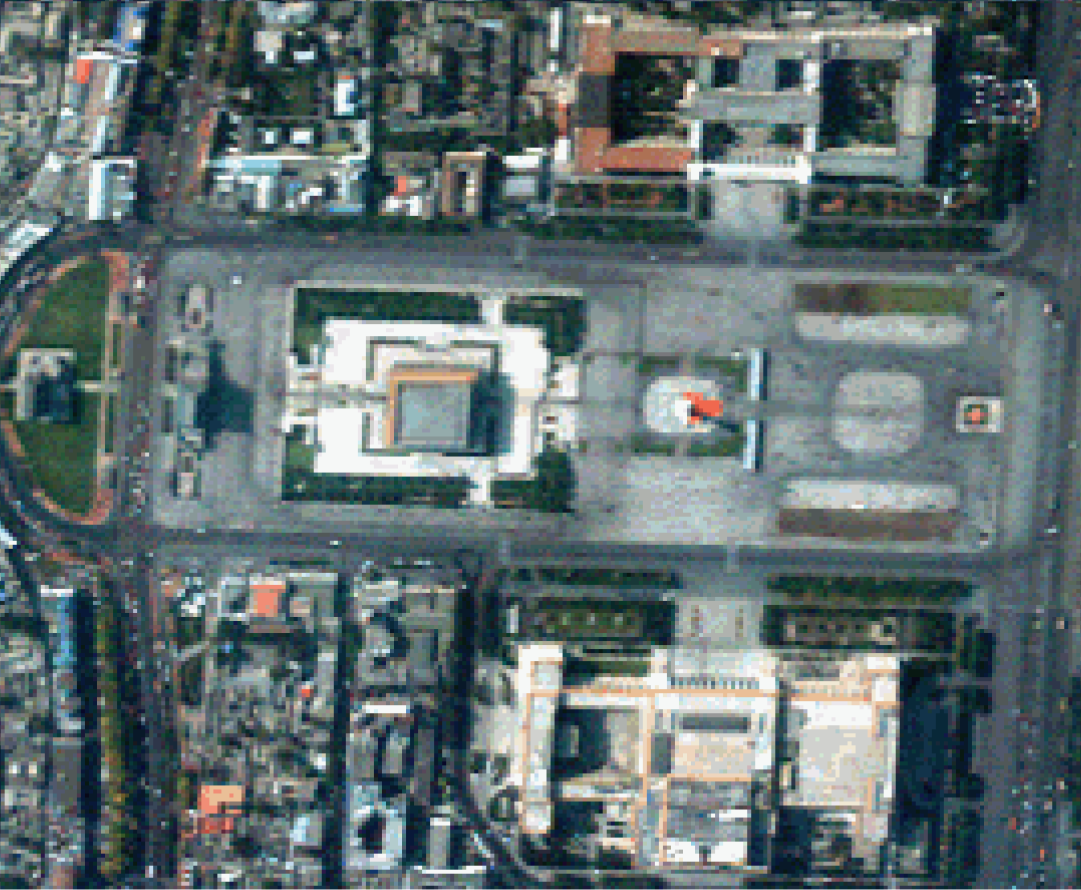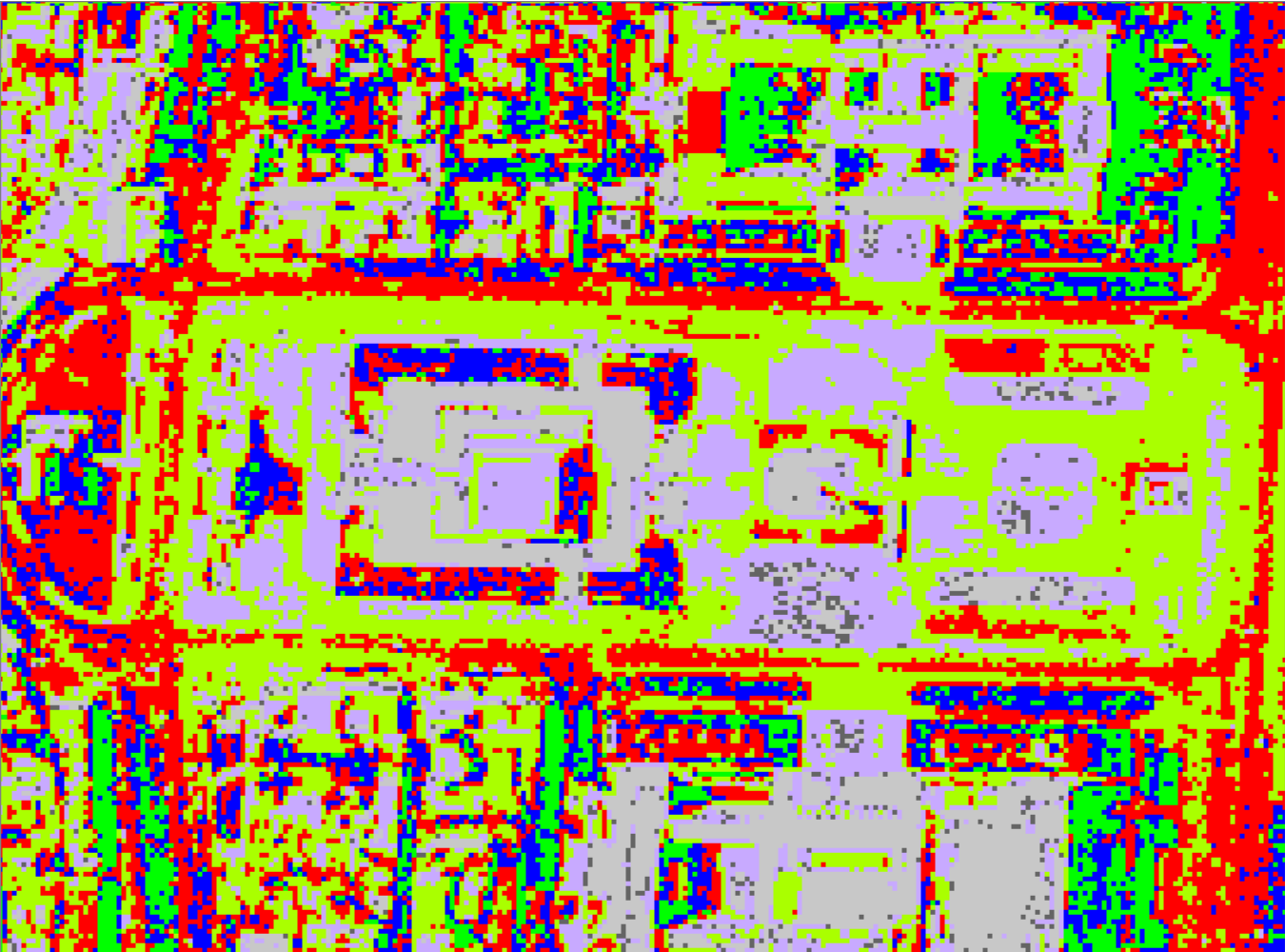分类效果图

k值 图像处理 相关内容

2019-10-22 16:05:53 weixin_43031412 阅读数 37
• ###### 用OpenStack和Kubernetes构建容器和虚拟机组合云平台

用OpenStack和Kubernetes构建容器和虚拟机组合云平台视频教程：1.集群的创建 2.容器和虚拟机网络问题 3.统一的身份认证 4.轻元科技在k8s上的创新和容器的存储方案

5829 人正在学习 去看看 CSDN讲师

scipy.ndimage中提供了图像矩阵变换、图像滤波、图像卷积等功能。

## 旋转图片

from scipy import ndimage
import matplotlib.image as mpimg
import matplotlib.pyplot as plt

# 加载图片

# 显示图片
plt.imshow(face)
# plt.savefig('./img2-1.png') # 保存要显示的图片
plt.show()


from scipy import ndimage
import matplotlib.image as mpimg
import matplotlib.pyplot as plt

# 加载图片

# 旋转图片
rotate_face = ndimage.rotate(face, 45)

plt.imshow(rotate_face)
# plt.savefig('./img3-1.png') # 保存要显示的图片
plt.show()


## 图像滤波

from scipy import ndimage
import matplotlib.image as mpimg
import matplotlib.pyplot as plt

# 加载图片

# 处理图片
face1 = ndimage.gaussian_filter(face, sigma=3)

# 显示图片
plt.imshow(face1)
# plt.savefig('./img4-1.png') # 保存要显示的图片
plt.show()


sigma=3表示模糊程度为3，我们可以通过调整sigma值，来比较图像质量的变化。

## 边缘检测

• Sobel
• Canny
• Prewitt
• Roberts
• Fuzzy Logic methods

import scipy.ndimage as nd
import numpy as np

im = np.zeros((256, 256))
im[64:-64, 64:-64] = 1
im[90:-90,90:-90] = 2
im = nd.gaussian_filter(im, 8)

import matplotlib.pyplot as plt
plt.imshow(im)
# plt.savefig('./img5-1.png') # 保存要显示的图片
plt.show()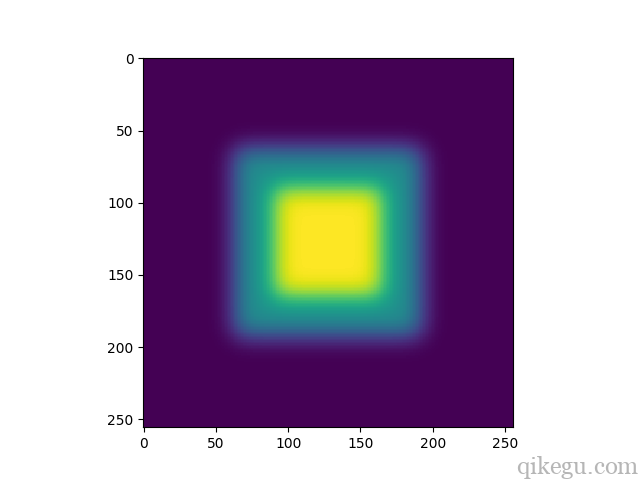import scipy.ndimage as nd
import numpy as np
import matplotlib.pyplot as plt

im = np.zeros((256, 256))
im[64:-64, 64:-64] = 1
im[90:-90,90:-90] = 2
im = nd.gaussian_filter(im, 8)

sx = nd.sobel(im, axis = 0, mode = 'constant')
sy = nd.sobel(im, axis = 1, mode = 'constant')
sob = np.hypot(sx, sy)

plt.imshow(sob)
# plt.savefig('./img6-1.png') # 保存要显示的图片
plt.show()


k值 图像处理 相关内容

2018-08-07 20:45:37 ChenQihome9 阅读数 1520
• ###### 用OpenStack和Kubernetes构建容器和虚拟机组合云平台

用OpenStack和Kubernetes构建容器和虚拟机组合云平台视频教程：1.集群的创建 2.容器和虚拟机网络问题 3.统一的身份认证 4.轻元科技在k8s上的创新和容器的存储方案

5829 人正在学习 去看看 CSDN讲师

# PART 1 导入图片并对图片进行取点操作

%% 建立主函数
function BTBU_mouse_track()  %BTBU_mouse_track() 运行时只要运行函数名即可
figure,imshow(in);
%% 显示坐标系，以及画平行于x轴与y轴的水平线，将图片分为四个区域
axis on;  % 打开（显示）坐标系
hold on;  plot([550,550],[0,1100],'r-'); %ylim 用于绘制y轴的取值范围
hold on;  plot([0,1100],[550,550],'r-'); %m-- 用于描述线型，粉色，虚线
BTBUbikedata = zeros(0,2);   %保存所取得点，并且以mat形式储存
save BTBUbikedata BTBUbikedata;
set(gcf,'WindowButtonDownFcn',@BTBU_ButttonDownFcn);

%% 回调函数
function [x,y]=BTBU_ButttonDownFcn(src,event)
pt = get(gca,'CurrentPoint');
x = pt(1,1);
y = pt(1,2);
BTBUbikedata(end+1,1)=round(y); %每次新产生的坐标加到BTBUbikedata中，注意figure中的x,y坐标跟实际的是反过来的
BTBUbikedata(end,2)=round(x);  %由于图像放大会产生非整数坐标，这里取整
[len,~] = size(BTBUbikedata);
fprintf('单车编号=%d,x=%f,y=%f\n',len,y,x);
save BTBUbikedata BTBUbikedata;

# PART 2 对数据进行K值聚类

load('BTBUbikedata.mat');  %% 导入数据
x=BTBUbikedata(:,2);       y=BTBUbikedata(:,1);
figure,imshow(in);         axis on;
hold on;  plot([550,550],[0,1100],'r-');   %ylim 用于绘制y轴的取值范围
hold on;  plot([0,1100],[550,550],'r-');   %m-- 用于描述线型，粉色，虚线
hold on;  plot(x,y,'ro')； xlim([0,1100]); ylim([0,1100]);%对X轴和Y轴设定显示范围
title('研究样本散点分布图')

[cidx2,cmeans2,sumd2,D2] = kmeans(BTBUbikedata,2,'dist','sqEuclidean'); %二类K值
P2 = figure;clf;
[silh2,h2] = silhouette(BTBUbikedata,cidx2,'sqeuclidean');
eucD = pdist(BTBUbikedata,'euclidean'); %分层聚类
cophenet(clustTreeEuc,eucD);
P3 = figure;clf;
[h,nodes] =  dendrogram(clustTreeEuc,20);
set(gca,'TickDir','out','TickLength',[.002 0],'XTickLabel',[]);  % 尝试用三类K值聚类分析
[cidx3,cmeans3,sumd3,D3] = kmeans(BTBUbikedata,3,'dist','sqEuclidean');
P4 = figure;clf;
[silh3,h3] = silhouette(BTBUbikedata,cidx3,'sqeuclidean');

# PART 3 对数据进行可视化操作

A=xlsread('BTBUbikedaily0801.xls');  %读取Excel表格数据
x=A(:,2); y=A(:,1);
x1=A(:,4); y1=A(:,3);
x2=A(:,6); y2=A(:,5);
x3=A(:,8); y3=A(:,7);
in = imread('图片.tif');   figure,imshow(in);    axis on;
hold on;  p1=plot([550,550],[0,1100],'r-'); %ylim 用于绘制y轴的取值范围
hold on;  p2=plot([0,1100],[550,550],'r-'); %m-- 用于描述线型，粉色，虚线
hold on;  p3=plot(x,y,'yo','MarkerFaceColor','y');   %绘制x和y的图像
hold on； p4=plot(x1,y1,'ro','MarkerFaceColor','r'); %MarkerFaceColor表示实心点
hold on； p5=plot(x2,y2,'bo','MarkerFaceColor','b');
hold on； p6=plot(x3,y3,'go','MarkerFaceColor','g');
legend([p3,p4,p5,p6],'昨日时间段03','时间段01','时间段02','时间段03','Location','northoutside','Orientation','horizontal');  %图例,注意图例会展示展示出所有前面plot所画的图像，包括所画的直线，因此采用[pi,pn]来限制图例的展示
title(legend,'0801各个时间段');    xlim([0,1100]);  ylim([0,1100]); %对X和Y轴设定显示范围
set(gca,'YDir','reverse');        %将x轴方向设置为反向(从上到下递增)。
title('显示同一天的三个时间段样本散点分布图');

——Written in BTBU

k值 图像处理 相关内容

2016-11-01 22:58:19 Serious_Tanx 阅读数 1583
• ###### 用OpenStack和Kubernetes构建容器和虚拟机组合云平台

用OpenStack和Kubernetes构建容器和虚拟机组合云平台视频教程：1.集群的创建 2.容器和虚拟机网络问题 3.统一的身份认证 4.轻元科技在k8s上的创新和容器的存储方案

5829 人正在学习 去看看 CSDN讲师

K均值聚类算法是先随机选取K个对象作为初始的聚类中心。然后计算每个对象与各个种子聚类中心之间的距离，把每个对象分配给距离它最近的聚类中心。聚类中心以及分配给它们的对象就代表一个聚类。一旦全部对象都被分配了，每个聚类的聚类中心会根据聚类中现有的对象被重新计算。这个过程将不断重复直到满足某个终止条件。终止条件可以是没有（或最小数目）对象被重新分配给不同的聚类，没有（或最小数目）聚类中心再发生变化，误差平方和局部最小。

（1） 从 n个数据对象任意选择 k 个对象作为初始聚类中心；

（2 ) 根据每个聚类对象的均值（中心对象），计算每个对象与这些中心对象的距离；并根据最小距离重新对相应对象进行划分；

（3） 重新计算每个（有变化）聚类的均值（中心对象） 三、代码(附说明)

（4）循环（2）到（3）直到每个聚类不再发生变化为止

// KmeanImage.cpp : 定义控制台应用程序的入口点。
//作者：Serious_Tanx
//时间：2016.11.01
//题目：根据K均值方法对图像灰度值进行分类
//作用：可以根据聚类后的值，来计算得出后续二值化的阈值

#include "stdafx.h"
#include <iostream>
#include <fstream>
#include <vector>
#include <math.h>

#define k 3  //分成3类

using namespace std;

struct Tuple //结构体的好处不用多说
{
float gray;
};

void KMeans(vector<Tuple>tuples);
Tuple getMeans(vector<Tuple>cluster);
float getVar(vector<Tuple>clusters[],Tuple means[]);
int clusterOfTuple(Tuple means[],Tuple tuple);
float getDistXY(Tuple t1,Tuple t2);

int _tmain(int argc, _TCHAR* argv[])
{
char fname;
cout<<"请输入存放数据的文件名：";
cin>>fname;
cout<<endl;
ifstream infile;
infile.open(fname,ios::in);
if(!infile)
{
cout<<"不能打开输入的文件"<<fname<<endl;
return 0;
}

int count=0;
vector<Tuple>tuples;//建立储存数据的容器
Tuple tuple;
while(!infile.eof())//读入数据
{
count++;

infile>>tuple.gray;
tuples.push_back(tuple);

}

for(vector<Tuple>::size_type ix=0;ix!=tuples.size();++ix)
cout<<tuples[ix].gray<<" ";
cout<<endl;

KMeans(tuples);
system("PAUSE");
return 0;
}

void KMeans(vector<Tuple>tuples)
{
vector<Tuple>clusters[k];

Tuple means[k];//质心，也就是均值

//默认一开始将前k个元祖的值作为k个簇的质心(均值)
for(int i=0;i<k;i++)
{
means[i].gray=tuples[i].gray;
}

int lable=0;

//根据默认的质心给簇赋值
for(int i=0;i!=tuples.size();++i)
{
lable=clusterOfTuple(means,tuples[i]);
clusters[lable].push_back(tuples[i]);
}

//输出刚开始的簇
for(lable=0;lable<k;lable++)
{
cout<<"第"<<lable+1<<"个簇:"<<endl;
vector<Tuple>t=clusters[lable];
for(int i=0;i<t.size();i++)
{
cout<<t[i].gray<<" ";
}
cout<<endl;
}

float oldVar=-1;
float newVar=getVar(clusters,means);

while(abs(newVar - oldVar) >=1)
{
for(int i=0;i<k;i++)
{
means[i] = getMeans(clusters[i]);
}
oldVar = newVar;
newVar = getVar(clusters,means);//计算新的准则函数值
for(int i=0;i<k;i++)
{
clusters[i].clear();
}
//根据新的质心获得新的簇
for(int i=0;i!=tuples.size();++i)
{
lable=clusterOfTuple(means,tuples[i]);
clusters[lable].push_back(tuples[i]);
}
//输出当前的簇
for(lable=0;lable<k;lable++)
{
cout<<"第"<<lable+1<<"个簇:"<<endl;
vector<Tuple>t=clusters[lable];
for(int i=0;i<t.size();i++)
{
cout<<t[i].gray<<" ";
}
cout<<endl;
}
}
}

//获得当前簇的均值(质心)
Tuple getMeans(vector<Tuple>cluster)
{
int num = cluster.size();
double meansX=0;
Tuple t;
for(int i=0;i<num;i++)
{
meansX +=cluster[i].gray;
}
t.gray = meansX/num;

return t;
}

//获得给定簇集的平方误差
float getVar(vector<Tuple>clusters[],Tuple means[])
{
float var=0;
for(int i=0;i<k;i++)
{
vector<Tuple> t=clusters[i];
for(int j=0;j<t.size();j++)
{
var +=getDistXY(t[j],means[i]);
}
}
return var;
}

//根据质心，决定当前元组属于哪个簇
int clusterOfTuple(Tuple means[],Tuple tuple)
{
float dist=getDistXY(means,tuple);
float tmp;
int label=0;
for(int i=1;i<k;i++)
{
tmp=getDistXY(means[i],tuple);
if(tmp<dist)
{
dist=tmp;
label=i;
}
}
return label;
}

//计算两个元组间的欧几里得距离
//注意，这里的是欧几里得距离，对于灰度值来说，就是灰度值之间的差或者差的平方根
float getDistXY(Tuple t1,Tuple t2)
{
//注意，这里为了数据更加好，可以加平方根
return abs(t1.gray - t2.gray);
}k值 图像处理 相关内容

2018-06-21 20:28:04 zx520113 阅读数 463
• ###### 用OpenStack和Kubernetes构建容器和虚拟机组合云平台

用OpenStack和Kubernetes构建容器和虚拟机组合云平台视频教程：1.集群的创建 2.容器和虚拟机网络问题 3.统一的身份认证 4.轻元科技在k8s上的创新和容器的存储方案

5829 人正在学习 去看看 CSDN讲师

5、图像滤波（平滑）

图像滤波（平滑），即在尽量保留图像细节特征的条件下对目标图像的噪声进行抑制，是图像预处理中不可缺少的操作，其处理效果的好坏将直接影响到后续图像处理和分析的有效性和可靠性。

常用的图像滤波算法高斯滤波、均值滤波、中值滤波、双边滤波等。

卷积的定义：假设被卷积的图像为I，卷积核为K。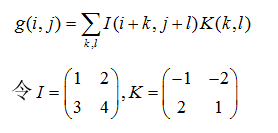I与K的二维离散卷积计算步骤如下：

首先将K翻转成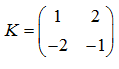，然后用K沿着I的每一个位置相乘求和，得到full卷积，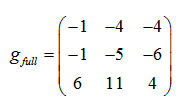，从filter和image刚相交开始做卷积。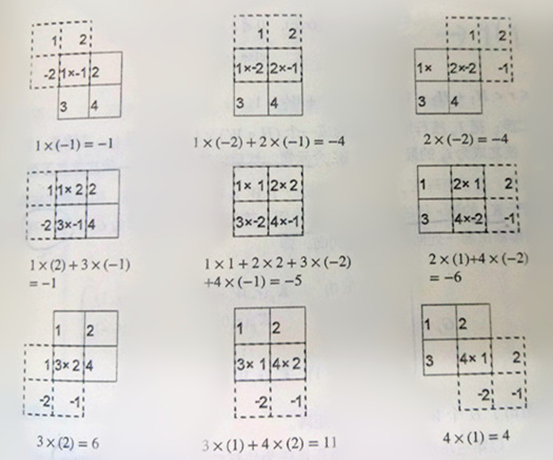valid卷积：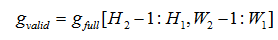当filter全部在image里面的时候，进行卷积运算。

same卷: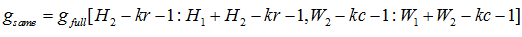当filter的中心(K)与image的边角重合时，开始做卷积运算。

在OpenCV中通过调用signal.convolve2d()实现卷积。

高斯滤波：高斯滤波（高斯平滑）是一种线性平滑滤波，适用于消除高斯噪声，广泛应用于图像处理的减噪过程。通俗的讲，高斯滤波就是对整幅图像进行加权平均的过程，每一个像素点的值，都由其本身和邻域内的其他像素值经过加权平均后得到。高斯平滑滤波器对于抑制服从正态分布的噪声非常有效。

高斯卷积算子的构建：

首先计算高斯矩阵：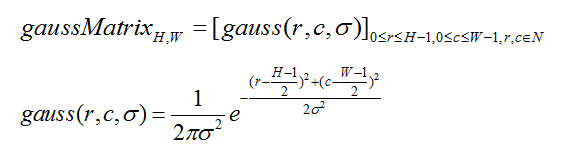再计算高斯矩阵的和：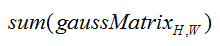最后用高斯矩阵除以本身的和，即归一化，得到高斯卷积算子：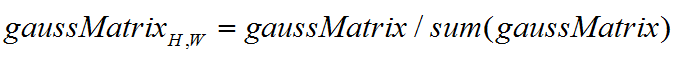在OpenCV中，通过调用cv2.getGaussianKernel(ksize,sigma,ktype)实现一维水平方向上的高斯平滑，再进行一维垂直方向上的高斯平滑。

均值平滑：均值滤波，是最简单的一种线性滤波操作，输出图像的每一个像素是核窗口内输入图像对应图像像素的平均值。其算法比较简单，计算速度快，但是均值滤波本身存在着固有的缺陷，即它不能很好地保护图像细节，在去除噪声的同时，也破坏了图像的细节部分，从而使图像变得模糊，不能很好地去除噪声点。但均值滤波对周期性的干扰噪声有很好的抑制作用。

均值平滑：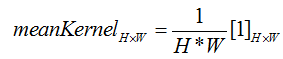利用矩阵积分，计算出矩阵中任意矩形区域内的和，快速均值平滑：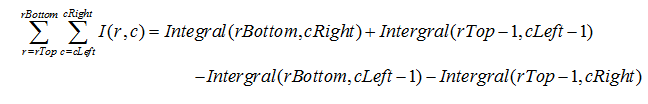在OpenCV中，通过调用cv2.blur()实现均值滤波。

中值滤波：中值滤波法是一种非线性平滑技术，将图像的每个像素用邻域 (以当前像素为中心的正方形区域)像素的中值代替 ，常用于消除图像中的椒盐噪声。中值滤波对脉冲噪声有良好的滤除作用，特别是在滤除噪声的同时，能够保护信号的边缘，使之不被模糊，但它会洗去均匀介质区域中的纹理。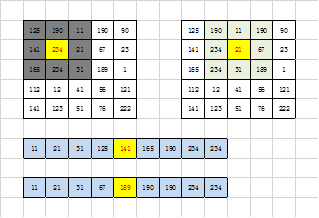再OpenCV中，通过调用cv2.medianBlur()实现中值滤波。

双边滤波：双边滤波（Bilateral filter）是一种非线性的滤波方法，是结合图像的空间邻近度和像素值相似度的一种折衷处理，同时考虑空域信息和灰度相似性，达到保边去噪的目的，具有简单、非迭代、局部的特点，能够对低频信息进行较好的额滤波。双边滤波器的好处是可以做边缘保存，这个特点对于一些图像模糊来说很有用。

双边滤波根据每个位置的领域，对应该位置构建不同的权重模板，首先，构建winH*winW的空间距离权重模板，与构建高斯卷积核的过程类似，winH*winW均为奇数，0<=h<winH,0<=w<winW。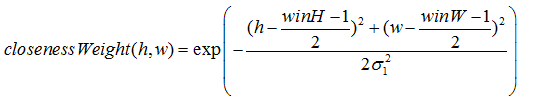然后，构建winH*winW的相似权重模板，是通过（r，c）处的值与其领域值得差值的指数衡量。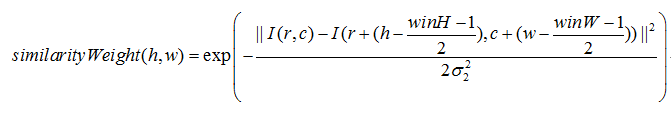最后将closenessWeight和similarityWeight的对应位置相乘，然后进行归一化，便可得到改位置的权重模板，将所得到的权重模板和该位置领域的对应位置相乘求和，最后就得到该位置的输出值。

在OpenCV中，通过调用cv2.bilateralFilter()实现双边滤波。

联合双边滤波与双边滤波不同之处在于，双边滤波是根据原图对每一个位置，通过该位置和其领域的灰度值的差的指数来估计相似性；而联合双边滤波是首先对原图进行高斯平滑，根据平滑的结果，用当前的位置及其领域的值得差来估计相似性权重模板。

k值 图像处理 相关内容